# For Loop in C#

## For Loop in C# with Examples

In this article, I am going to discuss For Loop in C# Language with Examples. Please read our previous articles, where we discussed Do While Loop in C# with Examples. At the end of this article, you will understand what for loop is and when and how to use for loop in C# Language with Examples.

##### For Loop in C#:

For loop is one of the most commonly used loops in the C# language. If we know the number of times, we want to execute some set of statements or instructions, then we should use for loop. For loop is known as a Counter loop. Whenever counting is involved for repetition, then we need to use for loop.

Let’s take an example and try to understand what it means by counting. Suppose you are preparing coffee. You don’t know how to prepare coffee; somebody has given you instructions and you’re following them. You are making coffee for 4 people.

Now the question is how much sugar you should add? You don’t know exactly how much sugar you need to add. So, what you will do, you will add some sugar and mix it and check whether the coffee is sweet, if it is not sweet enough, then again you will add some sugar and mix, and so on until the coffee becomes sweet enough. So, how many times you should add sugar to coffee? It has no fixed answer because you will add sugar to coffee until it becomes sweet enough. So, this is not based on counting. This is based on condition. The condition is until and unless the coffee is not sweet enough, you continue adding sugar and mix it.

Now somebody gives you instructions that add 4 tablespoons of sugar to make the coffee for four people. So, this is based on counting. There are many examples in daily life. We do things a fixed number of times. So, when you have to repeat the steps based on counting, then you need to use for loop.

##### For Loop Flowchart

The following diagram shows the flowchart of the for loop.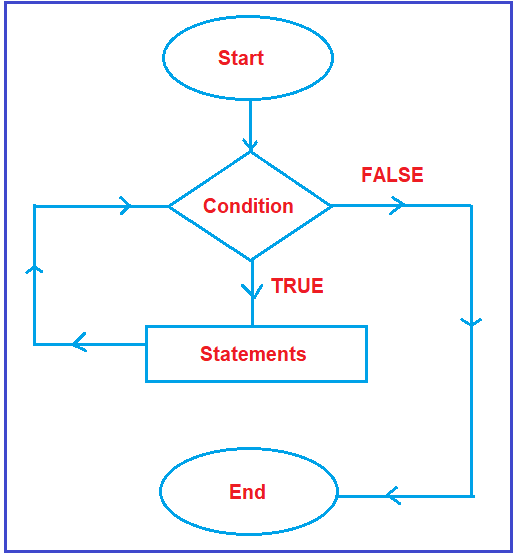The flow chart will start. The start is represented by the oval symbol. Then it will check the condition. As discussed earlier, every condition is having two outputs i.e. true and false. If it is true what will happen and it is false what will happen, we need to check.

Suppose, the condition is true, then all statements defined inside the block (within the for-loop block) will execute. After execution of statements, will it end? No, it will not end. After execution of statements, once again it will go and check the for-loop condition. It will repeat the same process as long as the given loop condition is true. And when the condition becomes false, then it will come to end. This is the execution flow of for loop in C#.

##### Syntax to use For Loop in C# Language:

The for loop allows the execution of instructions for a specific amount of time. It has four stages.

1. Loop initialization
2. Condition evaluation
3. Execution of instruction
4. Increment/Decrement

Now let’s have a look at the for loop syntax: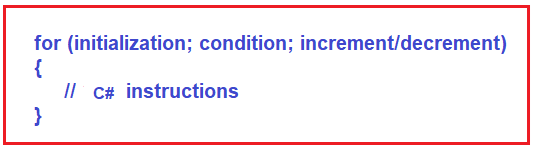###### Explanation of the for-loop syntax:
1. Loop Initialization: Loop initialization happens only once while executing the for loop, which means that the initialization part of for loop only executes once. Here, initialization means we need to initialize the counter variable.
2. Condition Evaluation: Conditions in for loop are executed for each iteration and if the condition is true, it executes the C# instruction and if the condition is false then it comes out of the loop.
3. Execution of Instruction: Once the condition is evaluated, and if the condition is true, then the control comes to the loop body i.e. the loop body is going to be executed.
4. Increment/Decrement: After executing the loop body, the increment/decrement part of the for loop will be executed, and once it executes the increment decrement part i.e. once it increments and decrements the counter variable, again it will go to the condition evaluation stage.
##### Points to Remember while working with for loop in C#:
1. When we are working with for loop always execution process will start from the initialization block. After the initialization block, the control will pass to the condition block. If the condition is evaluated as true then control will pass to the statement block.
2. After execution of the statement block, the control will pass to the increment/decrement statement, from the increment/decrement statement, it will pass back to the condition statement. Always repetition will happen beginning condition, statement block, and increment/decrement statement The initialization block will be executed only once when we are entering the loop for the first time.
3. When we are working with for loop everything is optional but mandatory to place 2 semicolons (;;).
4. While we are working with for loop if the condition part is not given then it will repeat infinite times because the condition part will replace it as non-zero. So it is always true as like for(; 1; )
5. In for loop also the pre-checking process will occur i.e. before the execution of the statement block (body of the for loop), the condition part will be executed.
##### Example to Print Numbers From 1 to n Using For Loop in C#:

First, we will take the input number from the user. This is the number up to which will print from one. Here, we will initialize the counter variable as 1 because we want to print the number from 1. Then we need to check whether the counter variable is less than equal to the input number which we have taken from the keyword/user. If the condition is true, then we will print the value of the counter, and then we will increase the counter variable by 1. Once we update the counter variable then we need to check the condition again and if the condition is true, we will repeat the same process. Once the condition becomes false, the loop will be stopped, and control will come out of the loop.

```using System;
namespace ControlFlowDemo
{
class Program
{
static void Main(string[] args)
{
Console.Write("Enter one Integer Number:");
for (int counter = 1; counter <= number; counter++)
{
Console.WriteLine(counter);
}
}
}
}
```
###### Output: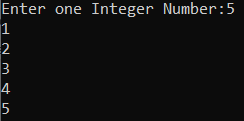In for loop, we can skip initialization, we can initialize a variable before for loop. So, Initialization is optional in for loop. Again, we can write the increment and decrement of the counter variable as part of the loop body as well. For a better understanding, please have a look at the following example where we initialize the counter variable before the loop and update the counter variable as part of the loop body. But remember, even if you have not specified the initialization and increment/decrement, you need to provide three statements in the for loop, in this case, you can simply write semicolons. The point is the second statement must be the condition.

```using System;
namespace ControlFlowDemo
{
class Program
{
static void Main(string[] args)
{
Console.Write("Enter one Integer Number:");
int counter = 1; //Initialization
for (;counter <= number;)
{
Console.WriteLine(counter);
counter++; //Updation
}
}
}
}```

You will get the same output as the previous example.

##### Infinite Loop in C#:

In our previous example, we have seen that we can place the increment/decrement statement in the body part. But what happens, if we don’t give any increment/decrement statement in for loop or in the body? It will be an infinite loop. An infinite loop is a never-ending loop. For a better understanding, please have a look at the below example. In the below example, we don’t have the increment/decrement statement, so for loop is not going to end and hence will result in an infinite loop.

```using System;
namespace ControlFlowDemo
{
class Program
{
static void Main(string[] args)
{
int number = 10;
int i = 1;
for (; i <= number; )
{
Console.WriteLine("Hello C#");
}
}
}
}
```
###### Output: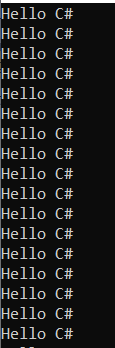You can see that it will go on printing “Hello C#” because here counter is not updated and the termination condition will never reach so it will continue printing “Hello C#” until you exit the program.

##### Can we run for loop without condition in C#?

Yes, we can run a for loop without condition. And, it will be an infinite loop. Because if we don’t mention any termination condition in for loop, the for loop is not going to end. For a better understanding, please have a look at the below example.

```using System;
namespace ControlFlowDemo
{
class Program
{
static void Main(string[] args)
{
for (int i = 1; ; i++)
{
Console.WriteLine("Hello C#");
}
}
}
}```
###### Output: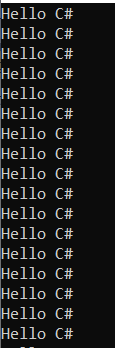So, it will be an infinite loop. Because this time we have written increment/decrement statement but haven’t mentioned any termination condition in for loop. So here ‘i’ value keeps increasing and printing “Hello C#”.

###### We can write a custom if statement inside for loop as:
```using System;
namespace ControlFlowDemo
{
class Program
{
static void Main(string[] args)
{
int i = 1;
for (;; )
{
Console.WriteLine("Hello C#");
i++;
if (i > 5)
break;
}
}
}
}
```
###### Output: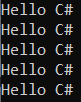##### Nested for Loop in C#:

When we created one for loop inside the body of another for loop, then it is said to be nested for loop in C# language. The syntax to use nested for loop is given below.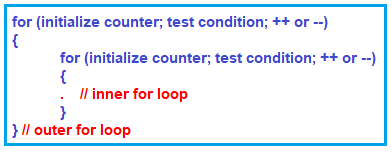Note: The point that you need to remember is when the inner for loop condition failed, then it will terminate the inner for loop only. And when the outer for loop condition failed, then it will terminate the outer for loop.

##### Example to Understand Nested For Loop in C#:

In the below example, we have created a nested for loop. The outer for loop is going to be executed 5 times and for each iteration of the outer for loop, the inner for loop is going to execute 10 times.

```using System;
namespace ControlFlowDemo
{
class Program
{
static void Main(string[] args)
{
for (int i = 1; i <= 5; i++) //Outer For Loop
{
Console.WriteLine(\$"Outer For Loop : {i}");
for (int j = 1; j <= 10; j++) //Inner For Loop
{
Console.Write(\$" {j}");
}
Console.WriteLine();
}

}
}
}
```
###### Output: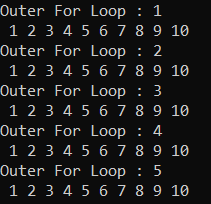#### For Loop Programs using C# Language:

Let us see some more examples using for loop in C# language.

##### Program to enter a number and check whether that no is the perfect number or not using for loop in C#

A perfect number is a positive integer that is equal to the sum of its positive divisors, excluding the number itself. For instance, 6 has divisors 1, 2 and 3, and 1 + 2 + 3 = 6, so 6 is a perfect number.

```using System;
namespace ControlFlowDemo
{
class Program
{
static void Main(string[] args)
{
int number, i, sum = 0;
Console.Write("Enter a Number :");

for (i = 1; i <= number / 2; i++)
{
if (number % i == 0)
sum += i;
}
if (sum == number && number != 0)
Console.WriteLine(\$"{number} is a Perfect Number");
else
Console.WriteLine(\$"{number} is not a Perfect Number");

}
}
}
```
###### Output: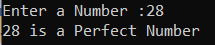##### Program to check whether a number is Armstrong number or not using for loop C# Language

An Armstrong Number is a number that is equal to the sum of, the power of each digit by the total number of digits. For example, the numbers such as 0, 1, 153, 370, 371, 407, 1634, 8208, 9474 are Armstrong numbers. Let us have a look at the following diagram which shows how the Armstrong number is calculated.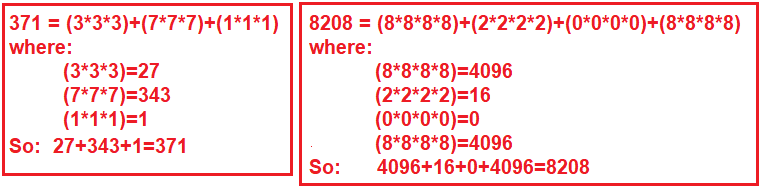```using System;
namespace ControlFlowDemo
{
class Program
{
static void Main(string[] args)
{
int i = 0;
int digitCount = 0;
int[] digitArray = new int;
double sum = 0;

//Step1: Take the input
Console.Write("Enter a Number : ");

//Step3: Store the number in a temporary variable
int temporaryNumber = number;

//Step3: Find the total number of digits in number as well as
//Store each each digit in the digit array
while (number > 0)
{
digitArray[i++] = number % 10;
number = number / 10;
digitCount++;
}

//Step4: Calculate the result
for (i = 0; i < digitCount; i++)
{
sum += Math.Pow(digitArray[i], digitCount);
}

//Step5: Check whether it is prime number or not
if (sum == temporaryNumber)
{
Console.WriteLine(\$"{temporaryNumber} is an Armstrong number");
}
else
{
Console.WriteLine(\$"{temporaryNumber} is not an Armstrong number");
}

}
}
}```
###### Output: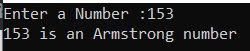For the Program explanation, please check the below article.

https://dotnettutorials.net/lesson/armstrong-number-program-in-csharp/

##### Program to enter a number and check whether it is a prime number or not using for loop in C# Language

A Prime Number is a number that should be greater than 1 and it is only divided by 1 and itself. In other words, we can say that the prime numbers can’t be divided by other numbers than itself and 1. For example, 2, 3, 5, 7, 11, 13, 17, 19, and 23…., are the prime numbers.

```using System;
namespace ControlFlowDemo
{
class Program
{
static void Main(string[] args)
{
int number, i;
Console.Write("Enter a Number :");

for (i = 2; i < number; i++)
{
if (number % i == 0)
{
break;
}

}
if (i == number && number >= 2)
{
Console.WriteLine(\$"{number} is a Prime Number");
}

else
{
Console.WriteLine(\$"{number} is not a Prime Number");
}

}
}
}```
###### Output: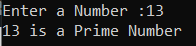##### Program to print the Fibonacci series up to a given number using for loop in C# Language
```using System;
namespace ControlFlowDemo
{
class Program
{
static void Main(string[] args)
{
int number, number1 = 0, number2 = 1, temp;
Console.Write("Enter a Number :");

if (number >= 1)
{
Console.Write(\$"{number1} {number2}");
temp = number1 + number2;

for (; temp <= number;)
{
Console.Write(\$" {temp}");
number1 = number2;
number2 = temp;
temp = number1 + number2;
}
}
else
Console.WriteLine("please enter a number greater than zero");

}
}
}
```
###### Output: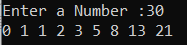Note: Foreach Loop in C# works with collections. So, we will learn for each loop once we learn array and collections in C#.

1.